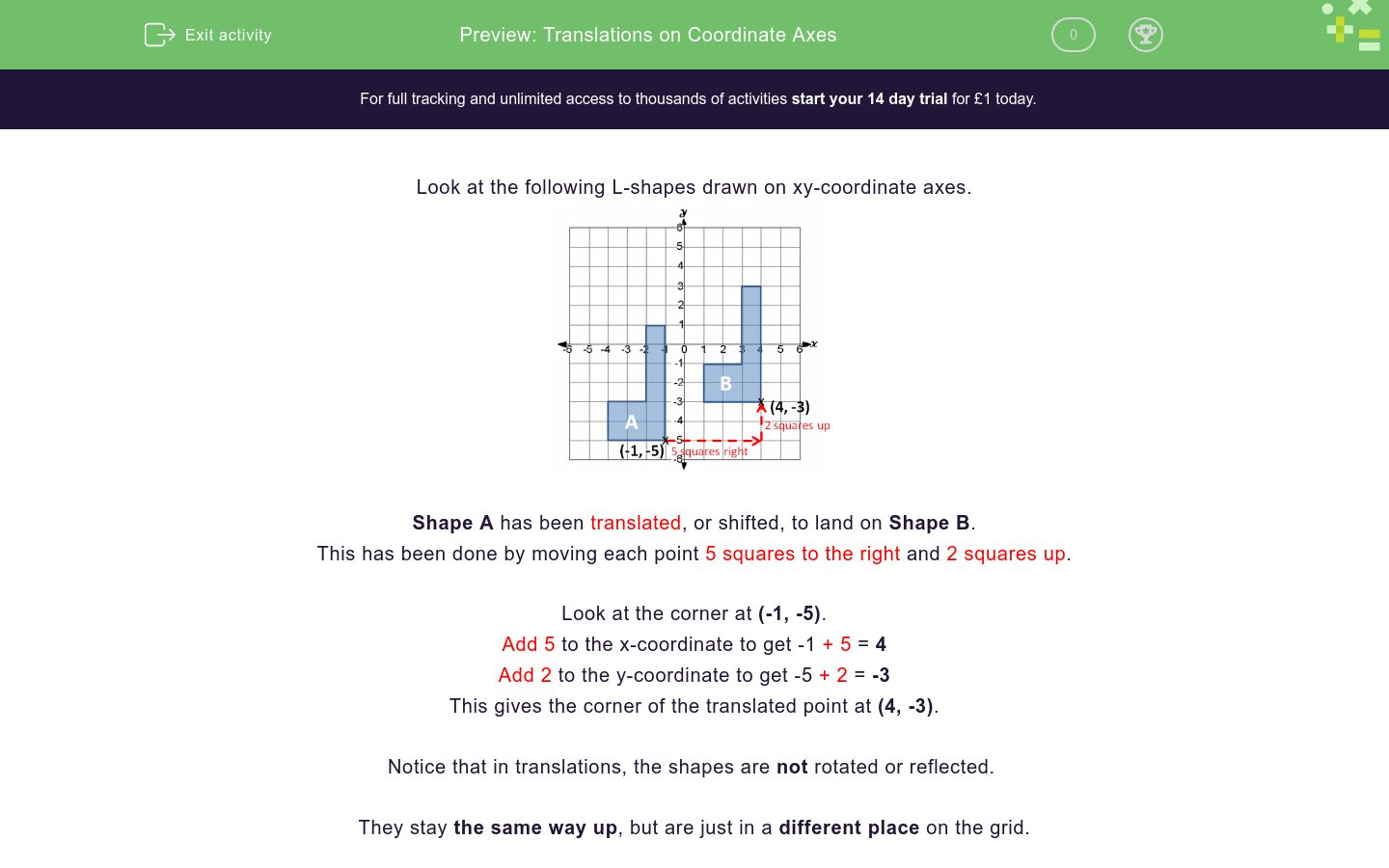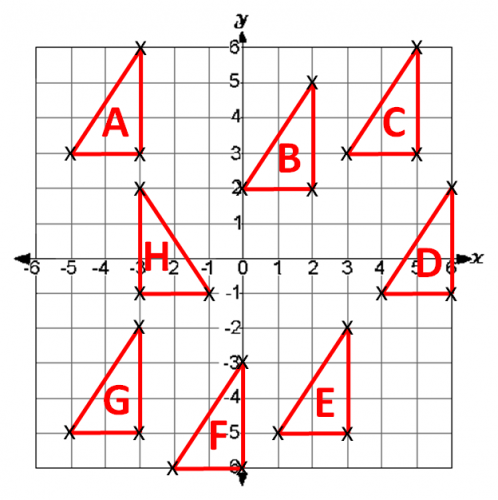# Translations on Coordinate Axes

In this worksheet, students translate shapes on a coordinate grid.Key stage:  KS 2

Curriculum topic:   Geometry: Position and Direction

Curriculum subtopic:   Draw/Translate Shapes

Difficulty level:### QUESTION 1 of 10

Look at the following L-shapes drawn on xy-coordinate axes.Shape A has been translated, or shifted, to land on Shape B.

This has been done by moving each point 5 squares to the right and 2 squares up.

Look at the corner at (-1, -5).

Add 5 to the x-coordinate to get -1 + 5 = 4

Add 2 to the y-coordinate to get -5 + 2 = -3

This gives the corner of the translated point at (4, -3).

Notice that in translations, the shapes are not rotated or reflected.

They stay the same way up, but are just in a different place on the grid.

Example

Look at the triangles on this coordinate grid.On which triangle does Triangle B land when it is translated:

3 squares to the right and 1 square up

Move each corner of Triangle B 3 squares to the right and 1 square up and it will land on:

Triangle C

Look at the triangles on this coordinate grid.

On which triangle does Triangle F land when it is translated:

3 squares to the right and 1 square upA

B

C

D

E

F

G

H

none of them

Look at the triangles on this coordinate grid.

On which triangle does Triangle E land when it is translated:

3 squares to the left and 1 square downA

B

C

D

E

F

G

H

none of them

Look at the triangles on this coordinate grid.

On which triangle does Triangle A land when it is translated:

5 squares to the right and 1 square downA

B

C

D

E

F

G

H

none of them

Look at the triangles on this coordinate grid.

On which triangle does Triangle F land when it is translated:

3 squares to the left and 1 square upA

B

C

D

E

F

G

H

none of them

Look at the triangles on this coordinate grid.

On which triangle does Triangle E land when it is translated:

6 squares to the left and 8 squares upA

B

C

D

E

F

G

H

none of them

Look at the triangles on this coordinate grid.

On which triangle does Triangle D land when it is translated:

3 squares to the left and 4 squares downA

B

C

D

E

F

G

H

none of them

Look at the triangles on this coordinate grid.

On which triangle does Triangle C land when it is translated:

1 square to the right and 4 squares downA

B

C

D

E

F

G

H

none of them

Look at the triangles on this coordinate grid.

On which triangle does Triangle H land when it is translated:

1 square to the right and 4 squares downA

B

C

D

E

F

G

H

none of them

Look at the triangles on this coordinate grid.

On which triangle does Triangle D land when it is translated:

9 squares to the left and 4 squares downA

B

C

D

E

F

G

H

none of them

Look at the triangles on this coordinate grid.

On which triangle does Triangle G land when it is translated:

8 squares to the right and 5 squares upA

B

C

D

E

F

G

H

none of them

• Question 1

Look at the triangles on this coordinate grid.

On which triangle does Triangle F land when it is translated:

3 squares to the right and 1 square upE
EDDIE SAYS
Each corner moves 3 squares to the right and 1 up.
• Question 2

Look at the triangles on this coordinate grid.

On which triangle does Triangle E land when it is translated:

3 squares to the left and 1 square downF
EDDIE SAYS
Each corner moves 3 squares to the left and 1 down.
• Question 3

Look at the triangles on this coordinate grid.

On which triangle does Triangle A land when it is translated:

5 squares to the right and 1 square downB
EDDIE SAYS
Each corner moves 5 squares to the right and 1 down.
• Question 4

Look at the triangles on this coordinate grid.

On which triangle does Triangle F land when it is translated:

3 squares to the left and 1 square upG
EDDIE SAYS
Each corner moves 3 squares to the left and 1 up.
• Question 5

Look at the triangles on this coordinate grid.

On which triangle does Triangle E land when it is translated:

6 squares to the left and 8 squares upA
EDDIE SAYS
Each corner moves 6 squares to the left and 8 up.
• Question 6

Look at the triangles on this coordinate grid.

On which triangle does Triangle D land when it is translated:

3 squares to the left and 4 squares downE
EDDIE SAYS
Each corner moves 3 squares to the left and 4 down.
• Question 7

Look at the triangles on this coordinate grid.

On which triangle does Triangle C land when it is translated:

1 square to the right and 4 squares downD
EDDIE SAYS
Each corner moves 1 square to the right and 4 down.
• Question 8

Look at the triangles on this coordinate grid.

On which triangle does Triangle H land when it is translated:

1 square to the right and 4 squares downnone of them
EDDIE SAYS
Triangle H is the wrong way round. It doesn't translate to any of the other shapes.
• Question 9

Look at the triangles on this coordinate grid.

On which triangle does Triangle D land when it is translated:

9 squares to the left and 4 squares downG
EDDIE SAYS
Each corner moves 9 squares to the left and 4 down.
• Question 10

Look at the triangles on this coordinate grid.

On which triangle does Triangle G land when it is translated:

8 squares to the right and 5 squares upnone of them
EDDIE SAYS
Each corner moves 8 squares to the right and 5 up, but this does not land on any of the given shapes.
---- OR ----

Sign up for a £1 trial so you can track and measure your child's progress on this activity.

### What is EdPlace?

We're your National Curriculum aligned online education content provider helping each child succeed in English, maths and science from year 1 to GCSE. With an EdPlace account you’ll be able to track and measure progress, helping each child achieve their best. We build confidence and attainment by personalising each child’s learning at a level that suits them.

Get started